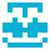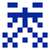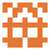# 2018.11 月赛题解

kblack edited 1 年，11 月前

kblack: Huge gap expected. （唔，好像真的惨案了）# Tag Idea Developer Tester
A 脑洞 改编自 GP of Shenyang 2018 I ultmaster oxx1108
B 模拟 ultmaster ultmaster kblack
C 几何 数学 oxx1108 ultmaster oxx1108
D 分类讨论 oxx1108 ultmaster zerol oxx1108
E 图论 DP oxx1108 ultmaster zerol
F 离散对数 分块 kblack kblack zerol

### Problem A

• 由于出题人是傻逼，TA 的做法是令后面四个数都是 0，然后前面两个数用 $2000 \times 2000$ 的暴力跑。跑完之后发现奇数都不行，然后枚举第三个数是 0 还是 1 就好了。
• 由于出题人是傻逼，这题锅了。

### Problem D

• $k \le n$，先猜至多 $k$ 次 1，由于回答 $<1$ 肯定是假的，所以可以把剩余系下是哪次错试出来，然后用至多 $n$ 次搞定。
• $k>n$，每个数都猜两次，如果两次结果不一样，再猜第三次确定哪个是真的，之后就可以当它一直说真话了（$n$ 次内不会再说假话）。

### Problem F

$$\begin{eqnarray} \frac {c(10^x-1)} 9 &\equiv& k &\pmod p \ c \cdot 10^x &\equiv& 9k+c &\pmod {9p} \ \end{eqnarray}$$

$$f(x) = f(x \bmod m) \times 10^{\lfloor\frac{x}{m}\rfloor \times m} + f(\lfloor\frac{x}{m}\rfloor \times m)$$

$$\begin{eqnarray} C \equiv 0 \pmod {\frac{p}{p’}} \ C \equiv f(x) \times 10^A \pmod{p’} \end{eqnarray}$$

// 看着整页的暴力好心痛。Problem D

k > n 时的另一个思路角度

• 按正常的二分执行，当成每次询问都是真话，记录每一次询问的结果：

• 如果中间等于了显然终止。
• 否则中间存在一个假话。
• 否则我们得到一个 < > 序列。

• 该序列有个特点，最后一段必然是 <>>>.... or ><<<...
• 原因是假话之后（相当于误导）会导致剩下的询问答案全为 > or <
• 所以可以借此找到上次假话，于是我们可以知道在这之后的每次询问是否为假话（因为隔K次一个假话）。

• run 一次二分。